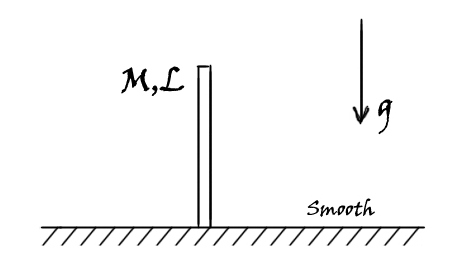# The Journey of the Solitary RodA uniform rod of mass $\displaystyle M$ and length $\displaystyle L$ is released from the position as shown. A slight disturbance causes the rod to start its journey to the floor.

When the rod makes an angle $\displaystyle \theta$ with the horizontal, find the angular speed of the rod (in $\displaystyle \frac{rad}{s}$), to the nearest integer.

Details and Assumptions:
$\bullet$ The floor is smooth and frictionless.
$\bullet$ $\displaystyle M = 10Kg$
$\bullet$ $\displaystyle L = 2m$
$\bullet$ $\displaystyle g = 9.8 m/s^2$
$\bullet$ $\displaystyle \theta = 30^o$

×